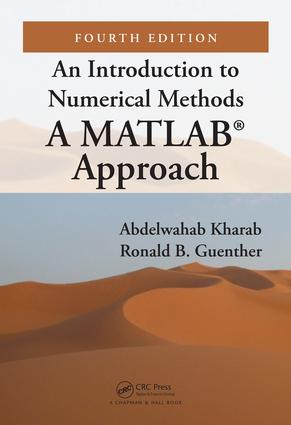# An Introduction to Numerical Methods

## A MATLAB® Approach, Fourth Edition, 4th Edition

CRC Press

615 pages | 133 B/W Illus.

##### Purchasing Options:\$ = USD
Hardback: 9781138093072
pub: 2018-07-10
\$99.95
x
eBook (VitalSource) : 9781315107042
pub: 2018-09-05
from \$49.98

FREE Standard Shipping!

### Description

Previous editions of this popular textbook offered an accessible and practical introduction to numerical analysis. An Introduction to Numerical Methods: A MATLAB® Approach, Fourth Edition continues to present a wide range of useful and important algorithms for scientific and engineering applications. The authors use MATLAB to illustrate each numerical method, providing full details of the computed results so that the main steps are easily visualized and interpreted. This edition also includes a new chapter on Dynamical Systems and Chaos.

### Reviews

Praise for Previous Editions

"Kharab and Guenther offer an attractive, clear, error-free, and well-written introduction to numerical methods … Highly recommended."

- J.H. Ellison, CHOICE

Praise for the New Edition

"An Introduction to Numerical Methods: A MATLAB Approach" provides a solid introduction to Numerical Methods. The book is easy to follow and most of the numerical methods are included making it ideal for students with little prior knowledge on the subject. The mathematical theories and explanations are quite easy to understand, several accompanying in-depth examples are provided, and most of the content is shared in a simple and direct manner.

Overall, this is one of the best numerical methods book that I have come across. I strongly recommend it to be used for any undergraduate introductory course in numerical analysis for science and engineering students."

-Jamal Benbourenane, Abu Dhabi University

### Table of Contents

1 Introduction

2 Number System and Errors

3 Roots of Equations

4 System of Linear Equations

5 Interpolation

6 Interpolation with Spline Functions

7 The Method of least-squares

8 Numerical Optimization

9 Numerical Diﬀerentiation

10 Numerical Integration

11 Numerical Methods for Linear Integral Equations

12 Numerical Methods for Diﬀerential Equations

13 Boundary-Value Problems

14 Eigenvalues and Eigenvectors

15 Dynamical Systems and Chaos

16 Partial Diﬀerential Equations

Bibliography and References

Appendix A Calculus Review

Appendix B MATLAB Built-in Functions

Appendix C Text MATLAB Functions

Appendix D MATLAB GUI

Answers to Selected Exercises

### About the Authors

Abdelwahab Kharab is an associate professor in the College of Arts and Sciences at Abu Dhabi University. His research interests include numerical analysis and simulation for the numerical solution of partial differential equations that arise in science and engineering.

Ronald B. Guenther is an Emeritus Professor in the Department of Mathematics at Oregon State University. His research interests include mathematically modeling deterministic systems and the ordinary and partial differential equations that arise from these models.

### Subject Categories

##### BISAC Subject Codes/Headings:
MAT000000
MATHEMATICS / General
MAT003000
MATHEMATICS / Applied
MAT021000
MATHEMATICS / Number Systems
TEC009070
TECHNOLOGY & ENGINEERING / Mechanical